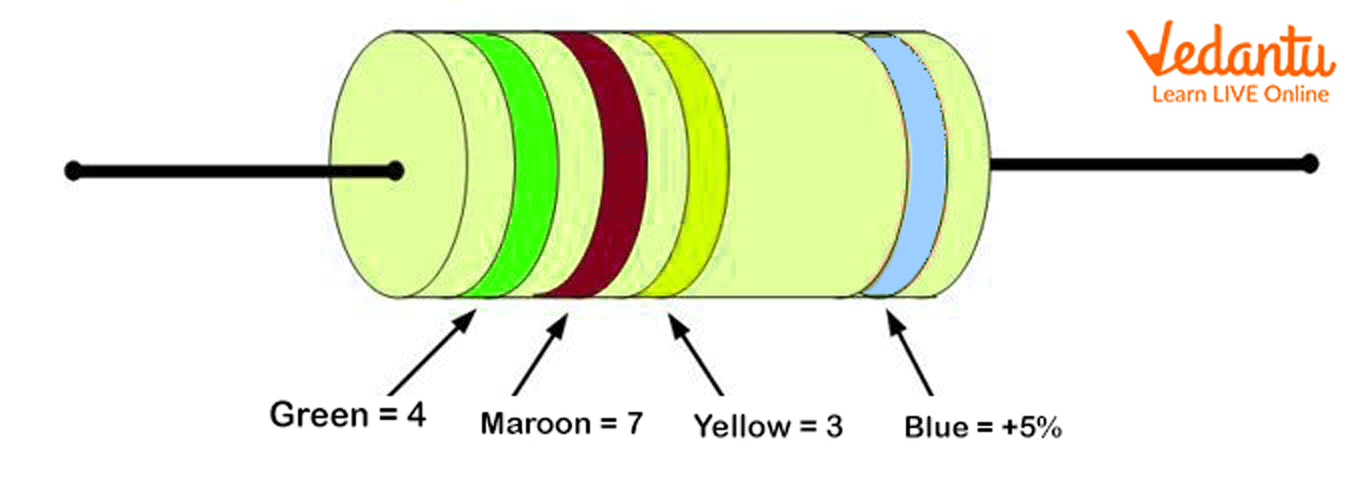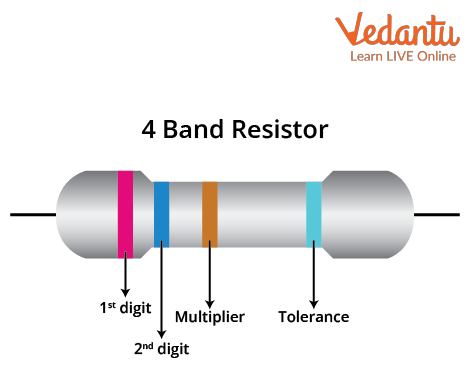Courses
Courses for Kids
Free study material
Free LIVE classes
More

# Colour Coding of Carbon Resistor for JEELIVE
Join Vedantu’s FREE Mastercalss

## What is the Colour Coding of a Carbon Resistor?

We know that resistors are passive elements  that are fundamental in forming the electrical circuit. Resistors are used to vary the magnitude of current through an electrical circuit. For easy calculation, resistors are available in different colour bands. There are four different colour bands available for resistors. Resistor colour codes are fundamental to knowing the value of resistors. In this article, we will learn why carbon resistors are colour coded and how to read them. We will also take a look at some examples of reading colour codes.

## Carbon Resistors and Their Colour Codes

Carbon resistors are made up of materials such as brass, platinum, tungsten, nichrome, etc. They are favoured in most of the applications as they are inexpensive, smaller in size, and can be directly printed on the circuit boards. Colour bands on resistors are printed on them to represent the electrical resistance.

Resistors with a power rating of one watt are marked with colour bands. The number of bands in a resistor varies from 3 to 6, where the first two bands indicate the value of the resistor and the third band indicates multipliers. The figure below denotes the different colour bands of a resistor. The colours such as  yellow, violet, orange, and gold are placed on the resistor.Different Colour Bands on a Resistor

Description: The image shows a carbon resistor with different colour bands.

From the figure above, we can see that the yellow colour code has a resistor value = 4, Violet = 7, the Orange colour code is 3, and the Gold colour code depicts a tolerance of 5 %.

## Resistor Colour Code Table

 Colour Band Resistance Colour Code Digit Multiplier Black B 0 100 Brown B 1 101 Red R 2 102 Orange O 3 103 Yellow Y 4 104 Green G 5 105 Blue B 6 106 Violet V 7 107 Grey G 8 108 White W 9 109

 Colour Multiplier Tolerance Gold 0.1 5% Silver 0.01 10% No colour 20%

## Reading the Resistor Colour Code and Its Mnemonic

It is easy to calculate the value of resistance if you know the different colour bands, its code, multiplier, and tolerance value. The reference for this can be taken from the table above.

First, the resistor should be held in a way that the tolerance value is to the right. It must be noted here that the tolerance values are usually denoted by the colours gold and silver and they are placed further apart from the other colour bands. Look at the figure below:Reading Colour Code on Carbon Resistor

So what does the ‘tolerance’ of a resistor mean?

The tolerance of the resistor is nothing but the error in the value of the resistor. It indicates the percentage difference between the actual value of the resistor and the value that is calculated. Thus, when the colour is gold, it denotes a tolerance value of 5%, whereas silver denotes a tolerance value of 10%.

Now, start from the left side of the resistor. It can be seen from the figure above that the first colour on the left-hand side is green. The second, third, and fourth colour bands are blue, black, and red, respectively. The colour band next to tolerance denotes the digit multiplier or in this case the colour code for red.

Using the table above, we can now note down the respective digit, the multiplier, and the tolerance value. So, for the figure above, the colour green has the value of 5, the blue colour code is denoted by digit 6, the black colour code is denoted by digit 0, the multiplier value for the colour red is 102 and the gold colour code is denoted by tolerance of $\pm 5\%$

The easiest way to remember the colour codes for the resistor is

Bill Brown Realised Only Yesterday Good Boys Value Good Work

OR

BB ROY of Great Britain had a Very Good Wife who wore Gold and Silver Necklace

The first letter of these words denote the colours of the bands on the resistor and also their digit value (except the position of the first colour begins with zero. Check the table above).  It is the quickest technique to find the resistance value of a particular resistor.

## Numerical Examples of Resistor Colour Code

Example 1: What colour bands would a resistor of resistance value 2000 Ω with a tolerance level $\pm 5\%$ have?

Solution:

The value of resistance with 2000$\pm 5\%$ denotes the colour bands (as seen from the table above):

Red-2

Black-0

Multiplier-100 (102) and

Gold- $\pm 5\%$

The multiplier will have a red colour.

So the colour band of the resistor will be red, black, red, gold.

Example 2: Find the value of a carbon resistor with colour bands orange, blue, brown, and gold.

Solution:

As we know, the first two colours depict significant digits of the resistance, the third colour is the multiplier and the last colour band is the tolerance value. As seen from the table above,

Orange colour code is:  3

Blue colour code is: 6

Brown colour code is: 101

And gold colour code is:  $\pm 5\%$

Therefore, the value of the resistor is 360$\pm 5\%$

## Conclusion

The article presents a thorough walkthrough of the colour-coding scheme for the carbon resistors. It also throws light on the importance of carbon resistance. The technique to find the resistance value of the carbon resistor with quick and easy ways to memorise the different colour bands and their values have also been explained in the article. Although resistors are passive elements, resistors play an important role in an electrical circuit. It controls the value of current in the circuit which entails an understanding and a measure of the value of resistors.

Last updated date: 16th Sep 2023
Total views: 132k
Views today: 1.32k

## FAQs on Colour Coding of Carbon Resistor for JEE

1. Why are only carbon resistors colour coded? How many colour bands are there?

Carbon resistors are colour coded as they are small in size. Thus, it is difficult to print the value of resistors on them. Therefore, to find out the value of resistors colour bands are printed on the resistors to find out the value of electrical resistance. Thus, the resistance colour coding scheme works well for the carbon resistors.  Additionally, colour bands are prioritised in many applications as it is easy to manufacture them, they are extremely small in size and can also be directly attached to the circuit board. There are three to six-colour bands on a resistor. In the six colour band resistors, the last colour band denotes the temperature coefficient.

2. Who invented the resistor colour code?

Developed by the Radio Manufacturers Association (RMA), the colour-coding scheme of resistors was developed in 1920. The RMA developed a three-band colour band for resistor value. It was the easiest and most compact way to find the value of the resistor as the third band depicted the number of zeroes. Initially, the tolerance or the fourth band was not available, it was added later. The colour bands had a completely different configuration earlier as there were no three separate bands and no neutral background colour. Also, adjacent colours were allowed.